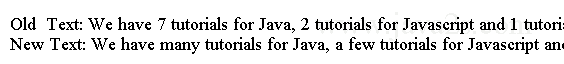# Java Regular Expression Tutorial - Java Regex Find/Replace

We can find a pattern and replace it with some text and the replaced text is depending on the matched text.

In Java we can use the following two methods in the Matcher class to accomplish this task.

```Matcher appendReplacement(StringBuffer sb, String replacement)
StringBuffer appendTail(StringBuffer sb)
```

## Example

Suppose we have the following text.

```We have 7 tutorials for Java, 2 tutorials for Javascript and 1 tutorial for Oracle.
```

We would like to change the number to text in the following rules.

• if more then 5, replace with many
• if less then 5, replace with a few
• if it is 1, replace with "only one"

After replacing, the above sentence would be

```We have many tutorials for Java, a few tutorials for Javascript and only one tutorial for Oracle.
```

To find all numbers, we can use `\b\d+\b`. `\b` marks the word boundaries.

The following code shows how to use Regular Expressions and appendReplacement() and appendTail() Methods

```import java.util.regex.Matcher;
import java.util.regex.Pattern;
/* ww  w .ja  v a  2 s.c  o m*/
public class Main {
public static void main(String[] args) {
String regex = "\\b\\d+\\b";
StringBuffer sb = new StringBuffer();
String replacementText = "";
String matchedText = "";

String text = "We have 7 tutorials for Java, 2 tutorials for Javascript and 1 tutorial for Oracle.";

Pattern p = Pattern.compile(regex);
Matcher m = p.matcher(text);

while (m.find()) {
matchedText = m.group();
int num = Integer.parseInt(matchedText);
if (num == 1) {
replacementText = "only one";
} else if (num < 5) {
replacementText = "a few";
} else {
replacementText = "many";
}
m.appendReplacement(sb, replacementText);
}

m.appendTail(sb);
System.out.println("Old  Text: " + text);
System.out.println("New Text: " + sb.toString());
}
}
```

The code above generates the following result.